#2018 iT 邦幫忙鐵人賽DAY 28
0
Software Development

# 擴展 Extensions

Swift 中的擴展可以：

• 添加計算實例屬性和計算類型屬性
• 定義實例方法和類型方法
• 提供新的初始化器
• 定義下標
• 定義和使用新的嵌套類型
• 使現有類型符合協議

## 擴展語法

``````extension SomeType {
// 添加新功能
}
``````

``````extension SomeType: SomeProtocol, AnotherProtocol {
// 協議要求的執行
}
``````

## 計算屬性

``````extension Double {
var km: Double { return self * 1000 }
var m: Double { return self }
var cm: Double { return self / 100 }
}
``````

``````let distance = 25.2345.km
let length = 123.4.cm
``````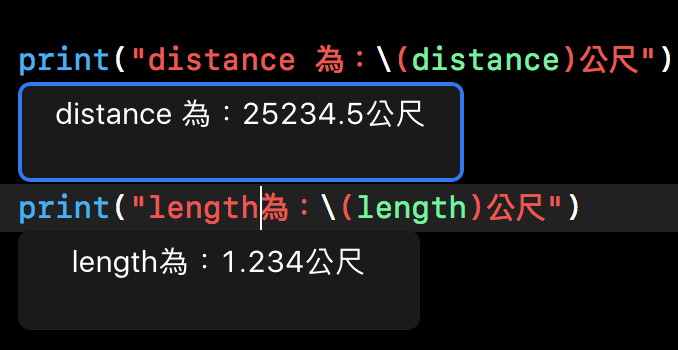``````let total = distance + length
let total2 = 123.km + 456.m
``````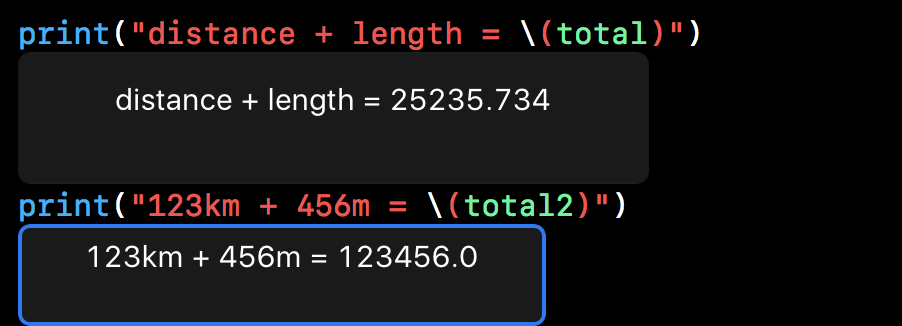## 初始化器

``````struct Player {
var name = "xxxxxx"
var coins = 100
}

extension Player {
init(name: String) {
self.name = name
}
}
``````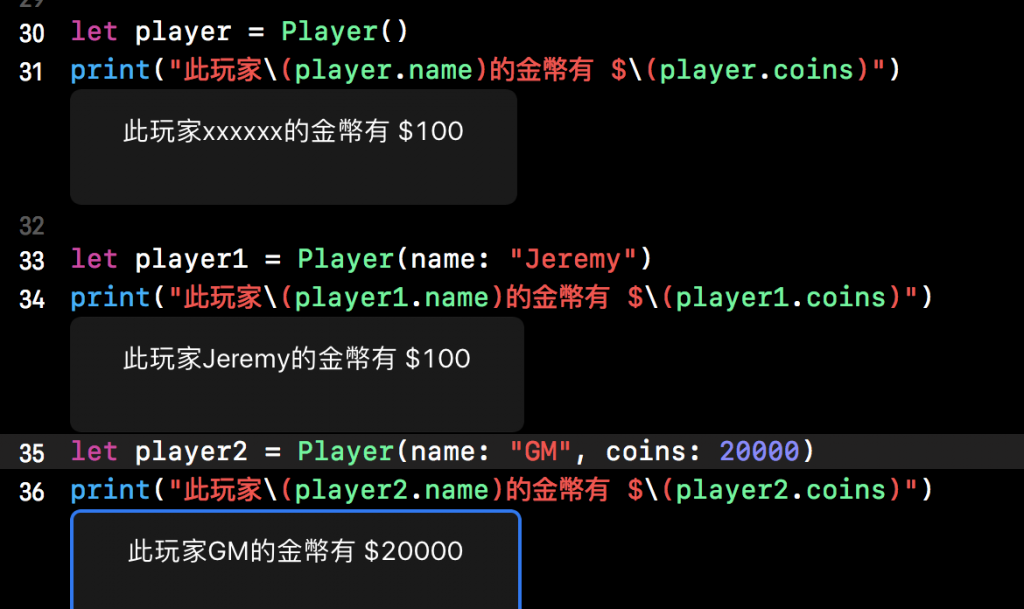## 方法

``````extension String{
func repeatPrint(_ number:Int) {
for _ in 0...number {
print(self)
}
}
}
``````

``````"hello".repeatPrint(3)
"IT鐵人說10次".repeatPrint(10)
``````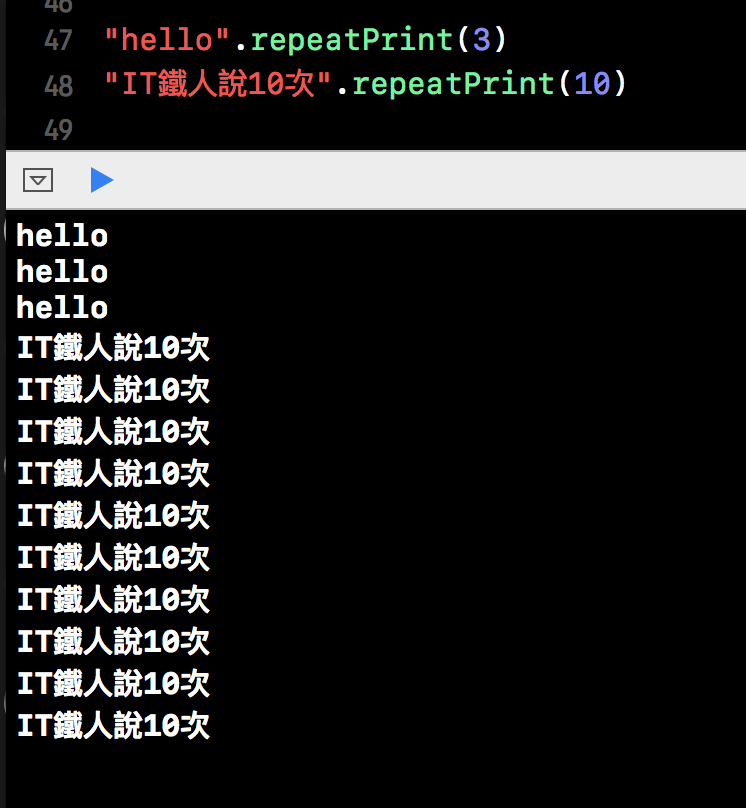## 異變實例方法

``````extension String{
mutating func hello(){
self = "Hello," + self
}
}

var name = "Jeremy"
name.hello()
``````## 下標

``````extension Int {
subscript(number: Int) -> String {
var decimal = 1
for _ in 1..<number {
decimal  *= 10
}
let result = (self / decimal ) % 10
if result != 0 {
return "從右開始算第\(number)個數字為\(result)"
} else {
return "超出數字範圍"
}
}
}
``````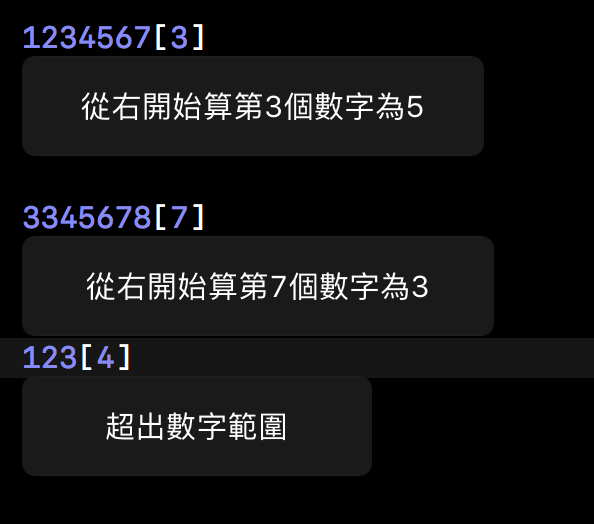## 內嵌類型

``````extension Int {
enum Kind {
case negative, zero, positive
}
var kind: Kind {
switch self {
case 0:
return .zero
case let i where i > 0:
return .positive
default:
return .negative
}
}
}
``````

``````func numberCheck(_ number:Int) {
switch number.kind{
case .positive:
print("\(number)是正數！")
case .zero:
print("它為的值 0。")
case .negative:
print("\(number)是負數！")
}
}
``````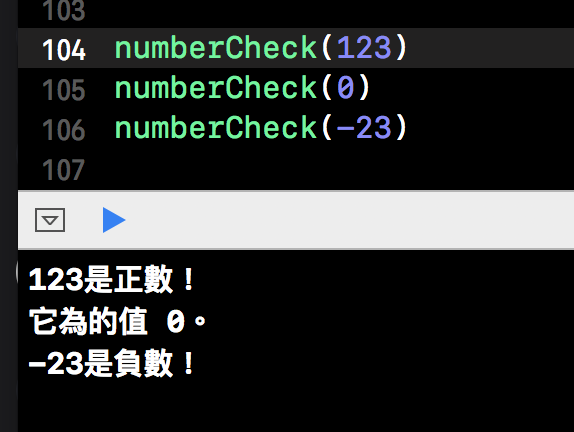Swift 菜鳥的30天30